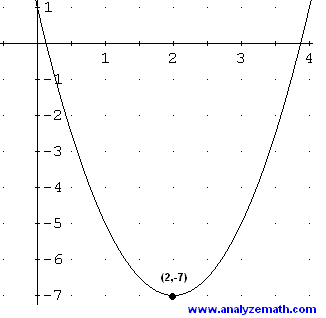## Composite & Inverse FunctionsBy the end of this topic you will:

• know domain, range, inverse, composite functions
• be able to find f(g(x)) given f(x) and g(x)

### 8 Responses

1. Wednesday 25 August, periods 5 & 6

Definition of a function, set notation, number sets, domain & range.

Substituting values and expressions into functions.

Composite functions.

Discuss targets one-one while class working through algebra.

Starter – Find the equations of these quadratic functions:2. Friday 27 August, period 5

Practice algebra for composite functions.
Class to create pairs of functions and calculate f(g(x)) and g(f(x)).

Due: Friday 3 September

3. Class worked well while I saw several pupils for 1-1 discussions on their targets.

Some further practice of trickier functions on Monday.

4. Monday 30 August, period 1

Starter: State the minimum value of the fraction${\frac{3}{6 - (x + 2)^2}}$

Further practice of composite functions, including fractional functions, three functions and problem solving.
Worksheet: Composite Functions

5. Very disappointing response to starter question.
A similar question will be posed tomorrow.

Most of worksheet completed – class to complete as part of their revision/practice during the week.

6. Tuesday 31 August, period 4

Return marked Algebraic Functions homework and discuss issues arising.
Remind class about self-assessment & next steps.
Some pupils will need to redo parts of this exercise.

Starter similar to previous lesson.

Inverse Functions
“If y = 3x – 1, what value of x gives y = -7?”

Define and create examples to calculate algebraically.

• Most of class missing due to Product Design trip.
Those present worked on introduction to inverse functions

7. Wednesday 1 September, periods 5 & 6

Return marked Algebraic Functions homework and discuss issues arising.
Remind class about self-assessment & next steps.
Some pupils will need to redo parts of this exercise.

Starter similar to previous lesson.

Inverse Functions
“If y = 3x – 1, what value of x gives y = -7?”

Define and create examples to calculate algebraically.